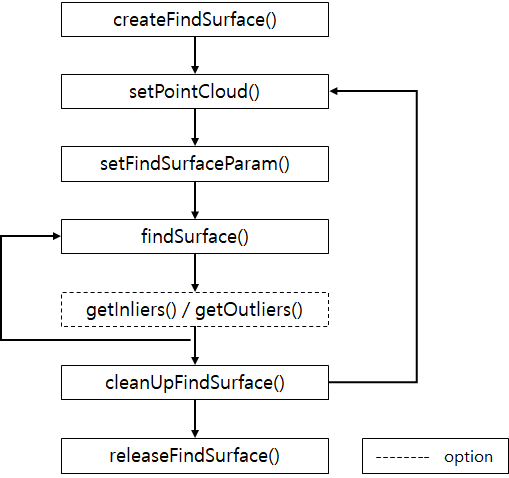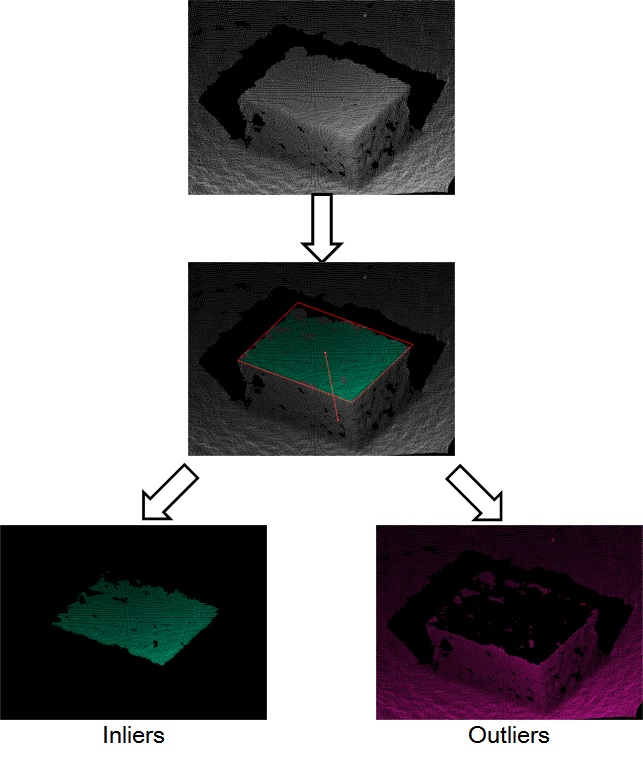# FindSurface Quick Start

FindSurface is a native-code API for analyzing raw point cloud data without any pre-processing like meshing, smoothing, and so on. This topic illustrates how to use CurvSurf 's FindSurface SDK.

General way to use FindSurface is like below flow-chart.## Example: Get a plane from the scene.

Below instruction shows how to extract a plane from the point cloud data.

### Step2: Create FIND_SURFACE_CONTEXT

Every FindSurface example creates a FIND_SURFACE_CONTEXT in a beginning.

``````FIND_SURFACE_CONTEXT ctx = NULL;
int ret = createFindSurface(&ctx);
``````

The FIND_SURFACE_CONTEXT is the starting point to use FindSurface SDK.
In general, your application should create a context once and retain it for the life of the application.

### Step3: Set Point Cloud Data

After you create a context, set the point cloud data to the context.

``````/* tightly packed float array  [ x1, y1, z1, x2, y2, z2, ... ] */
float *pPointCloudList;
int    nPointCloudCount;

/* Set Target Point Cloud Data */
int ret = setPointCloudFloat(ctx, pPointCloudList, nPointCloudCount, 0);
``````

Generally, FindSurface expects tightly packed float or double array for point cloud data. If you want to set custom type of point cloud data, you can handle it like below code sample.

``````/* First 3 components of custom type of the point cloud data must be x, y, z. */
typedef struct {
double        x, y, z;
int           intensity;
unsigned char r, g, b;
} MyPointCloud;

MyPointCloud *pPointCloudList;
int           nPointCloudCount;

/* Set Target Point Cloud Data */
int ret = setPointCloudDouble(ctx, pPointCloudList, nPointCloudCount, sizeof(MyPointCloud));
``````

### Step4: Set Parameters

Next step is setting algorithm parameters. At least 3 following parameters should be confirmed in your application.

1. measurement accuracy of input data.
2. mean distance of neighboring points.
3. touch radius that indicates the size of seed region.

measurement accuracy and mean distance are parameters that related to the measurement device. touch radius is parameter that related to the scale of target object.

Note If you want to get more information about these parameters, see Concepts topic.

You don't need to set these parameter values exactly. You can set the parameter values roughly. In most cases, it works fine unless the parameter values are too much lower than actual values. For example, if the accuracy of measurement device is about 3 mm, you can also set the measurement accuracy to 6 mm which is twice of the actual value.

Below code shows how to set the parameter values. We assume the measurement accuracy is about 3mm and the measurement unit is in meter. Also, we do not know exact value of neighboring points of mean distance.

``````/* Individual parameters depend on the Sensor used */

/* Set Sensor Measurement Accuracy */
setFindSurfaceParamFloat(ctx, FS_PARAM_ACCURACY, 0.003f);

/* Set Mean Distance of Neighboring Points */
/* Don't know exact value, so using a large enough value */
setFindSurfaceParamFloat(ctx, FS_PARAM_MEAN_DIST, 0.01f);

/* Touch Size of the Seed Region */
setFindSurfaceParamFloat(ctx, FS_PARAM_TOUCH_R, 0.05f);
``````

### Step5: Find a Plane

Now we are ready to find a plane from our point cloud data. All we need to do is to specify the feature type and the start point.

``````FS_FEATURE_RESULT result;
int start_index;
int ret = findSurface(ctx, FS_TYPE_PLANE, start_index, &result);

if (ret == FS_NO_ERROR) {
/* Succeed to find the plane. */
/*
* You can access the result parameter
* by using FS_FEATURE_RESULT structure.
* e.g. result.plane_param
*/
}
else {
/* Failed to find the plane */
}
``````

The 3rd parameter start_index is the index number of the seed point. The seed point is the interest point to recognize the specific surface.

The figure below shows the concept of the seed point and the seed region. (The magenta point in the figure is the seed point.)### Step6: Get Inliers or Outliers, If Necessary

You can get inliers and outliers after the specific surface is found. By using inliners information, you can calculate your own result object boundary. Or with outliers you can continue to find other features. This is useful when you find an object in entire scene by setting the seed point using random sampling.

``````float       *pOutBuf  = NULL;
unsigned int nBufSize = 0;

/* Get the total number of outliers */
unsigned int nCnt = getOutliersFloat(ctx, NULL, 0);
if(nCnt > 0) {
/*
* This function returns outliers as
* tightly packed array of x, y, z.
* So, minimum buffer size is
* (sizeof(float) * 3 * nCnt)
*/
nBufSize = sizeof(float) * 3 * nCnt;
pOutBuf = /* allocate memroy */;

getOutliersFloat(ctx, pOutBuf, nBufSize);
}
``````

The figure below shows the concept of the inliers and outliers.### Step7: Clean Up FIND_SURFACE_CONTEXT

If you want to continue FindSurface with new point cloud data. You have to clean up the context first, before to set the new point cloud data.

For example if you want to continue finding a plane from outliers, first clean up the context and then set the outliers as the target point cloud data. And then repeat from Step4 or Step5 to the current step.

``````/* Continued from the previous code */
nCnt = getOutliersFloat(ctx, pOutBuf, nBufSize);
/* Clean up the context first */
cleanUpFindSurface(ctx);
/* and set the outliers as the new target point cloud data */
setPointCloudFloat(ctx, pOutBuf, nCnt, 0);
``````

### Step8: Release FIND_SURFACE_CONTEXT

When your application using FindSurface has finished (i. e., when it is about to exit), release the FIND_SURFACE_CONTEXT.

``````releaseFindSurface(ctx);
``````

## Next Steps

In this page, you learned how to use FindSurface SDK. You can download full version of above example code from here. Also, you can find more examples from our GitHub page.